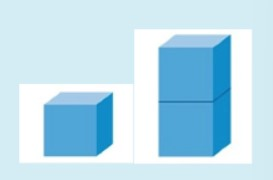# Dynamic Equilibrium 171

Some reactions occur in only one direction, for example, the combustion of a hydrocarbon. Once it is burned, that reaction cannot be reversed. Many reactions, however, are reversible, meaning that the products can react to form the reactants again. A common example of this is the physical change from liquid water to water vapor and back again. Not only can a reaction like this be reversible, both the forward and reverse reactions can happen at the same time. When this occurs at the same rate, we say that the system is in equilibrium. To indicate equilibrium, we use a double arrow in the equation.

H2O(l) ↔ H2O(g)

OR

H2O(l) ⇌ H2O(g)

In a closed container, as in the example below, these processes continuously occur. This is called dynamic equilibrium.In dynamic equilibrium, reactions continue to proceed in the forward and reverse direction; however, there is no net change in the amount of product or starting material.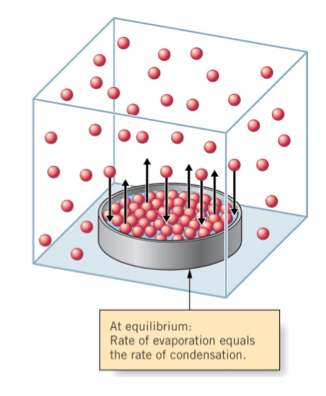Dynamic equilibrium occurs in chemical reactions as well. Consider this reaction in a closed container:

A ↔ B

When the reactants are placed in the container, their concentration is at its maximum. This concentration decreases as the product is formed. The concentration of the product begins at zero and increases as the reaction proceeds. Eventually the concentrations stop changing, even though the reaction is not complete. A state of dynamic equilibrium has been reached.

Equilibrium exists in a reaction when the concentrations of the reactants and products become constant. It appears from the outside that the reaction has stopped. In reality, the forward reaction and the reverse reaction, indicated by the double arrow, ⇌ , both still occur; they just occur at the same rates. It is a common misconception that the concentrations of reactants and products are equal at equilibrium. This is not true.

The change in concentration of reactants and products as the reaction proceeds is shown in this graph. Notice that the reactants concentration start at the maximum and the products concentration start at zero.

A ⇌ BEquilibrium is reached at the point indicated by the dotted line. Can you tell why the line is drawn at this particular place in the graph?

It is because this is the point where the concentrations of the reactants and products remain constant. Notice that their concentrations are not equal. That is not required for equilibrium.

The graph below shows the same reaction in reverse.

B ⇌ A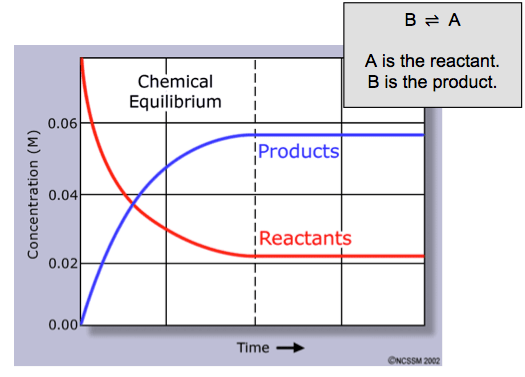Notice above that the concentration of A and B end up in the same position regardless of which one was the reactant and which was the product.

The concept of equilibrium can be confusing. Click on the link in the sidebar to an animation that can be used as an analogy for an equilibrium system.

## Equilibrium Law

There is a simple relationship among the concentrations of the reactants and products for any reversible chemical reaction. It is called the mass action expression, and is derived from thermodynamics. Consider the reversible reaction:

aA + bB ⇌ cC + dD

The mass action expression is: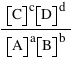Things to make note of in the mass action expression:

• The brackets [X] are hard brackets, not parenthesis. These mean the concentration of substance X in molarity.
• Molar concentrations of products are always placed in the numerator and reactants in the denominator.
• The concentrations are multiplied by each other, not added.
• The coefficients of the balanced equation become exponents.
• Solids and liquids are NOT included because their concentrations are not variable.

The numerical value of the mass action expression is called the reaction quotient and is symbolized by the letter, Q.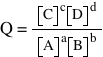When a reaction system reaches a state of equilibrium, Q is a constant called the equilibrium constant, represented by Kc. The equation above becomes: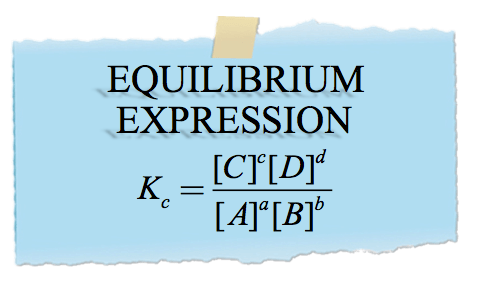Now, let’s calculate the value of Kc for a specific reaction.Now that you have determined Kc for this reaction, you can use it to determine if the reaction is in equilibrium when the concentrations are different. Here, we will calculate Q and compare it with Kc to see if the system is at equilibrium.Look at the picture of the solid cubes. Why do you think the concentration of a solid or liquid will be incorporated into the value of Kc?